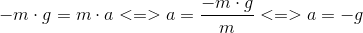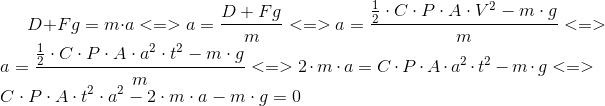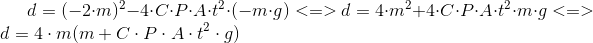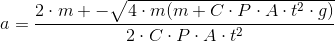# Air drag force: Why heavy objects accelerate faster?

babaliaris
I've done the math but because i can't find this answer anywhere in the book which I'm reading and on the web, I would like to be sure if my calculations are correct. I know that if two objects (starting at the same time) are free falling without a drag force being applied to them, the acceleration is:Which means it does not depend on the mass of the object. So the two objects no matter their mass, will accelerate with the same magnitude and they will reach the ground at the same time.

On Earth,if you see two objects free falling, the heavier one will accelerate faster. So let's see what maths can tell as by applying Newtons second law:

C = coefficient of air's drag force.
P = air's density
A = the area of the free falling body.
V = the velocity of the free falling body.
D = Drag force.
Fg = body's weight.

Be aware that we don't have an initial velocity.So if we assume that we are leaving two objects with the same Area (A) (a metal block and a leaf) and the metal's mass >> leafs mass , the above formula explains why the metal block will accelerate faster (numerator is big if m is big so does the acceleration).

By the way the maths which i did above, until today, i didn't know that you can use maths to explain things in such a way. I started reading classical physics 3 days ago and they already made me understand how you can use mathematics to explain things! Literally, i didn't know i could use maths in such a way! I only knew how to solve exercises and nothing more! I love physics!

#### Attachments

Last edited:
•Drakkith

•